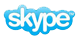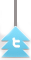## 2.1.1 Define displacement, velocity, speed and acceleration.

Displacement
Displacement is the distance moved in a particular direction. It is a vector quantity.

SI unit: m
Symbol: s

Velocity
Velocity is the rate of change of displacement. It is a vector quantity.
Velocity = (change in displacement / change in time)

SI unit: m s-1
Symbol: v or u

Speed
Speed is the rate of change of distance. It is a scalar quantity.
Speed = (change in distance / change in time)

SI unit: m s-1
Symbol: v or u

Note that speed and velocity are not the same thing. Velocity has a direction.

Acceleration
Acceleration is the rate of change of velocity. It is a vector quantity.
Acceleration = (change in velocity / change in time)

SI unit: m s-2
Symbol: a

Note that acceleration is any change in velocity, meaning an increase or decrease in velocity or a change in direction.

## 2.1.2 Explain the difference between instantaneous and average values of speed, velocity and acceleration.

Instantaneous
An instantaneous value of speed, velocity or acceleration is one that is at a particular point in time.

Average
An average value of speed, velocity or acceleration is one that is taken over a period of time.

## 2.1.3 Outline the conditions under which the equations for uniformly accelerated motion may be applied.

The equations of uniformly accelerated motion can only be under conditions where the acceleration is constant.

The equations of uniformly accelerated motion are as follows:

Table 1.2.1 - Variables used in uniformly accelerated motion equations
Variable Symbol
t time taken
s distance travelled
u initial velocity
v final velocity
a accelerationOther equations may be derived from these equations.

## 2.1.4 Identify the acceleration of a body falling in a vacuum near the Earth's surface with acceleration g of free fall.

When we ignore the effect of air resistance on an object falling down to earth due to gravity we say the object is in free fall. Free fall is an example of uniformly accelerated motion as the only force acting on the object is that of gravity.

On the earths surface, the acceleration of an object in free fall is about 9.81 ms-1. We can easily recognise the uniform acceleration in displacement - time, velocity - time and acceleration - time graphs as shown below:

## 2.1.5 Solve problems involving the equations of uniformly accelerated motion.

A car accelerates with uniformly from rest. After 10s it has travelled 200 m.

Calculate:

Its average acceleration

S = ut + 1/2 at²

200 = 0 x 10 +  1/2  x a x 10²

200 = 50a

a = 4 m s-2

Its instantaneous speed after 10s

v² = u ² + 2as

= 0 + 2 x 4 x 10

= 80

V= 8.9 m s-1

## 2.1.6 Describe the effects of air resistance on falling objects.

Air resistance eventually affects all objects that are in motion. Due to the effect of air resistance objects can reach terminal velocity. This is a point by which the velocity remains constant and acceleration is zero.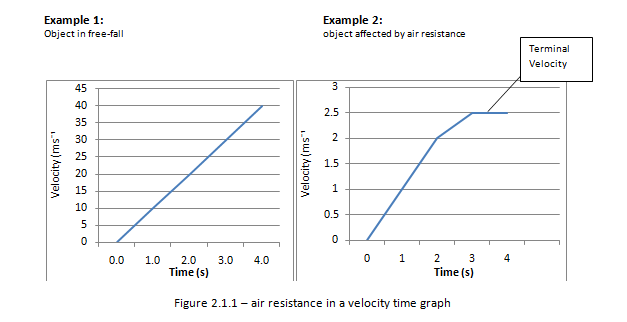Figure 2.1.1 – Air resistance in a velocity time graph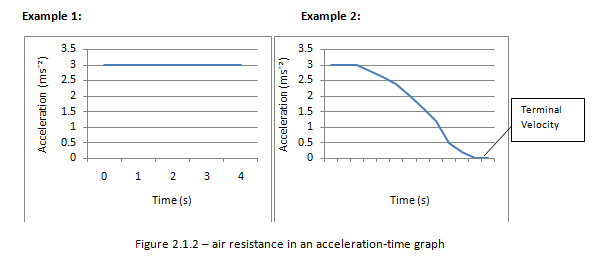Figure 2.1.2 – Air resistance in an acceleration-time graph

In the absence of air resistance all objects have the same acceleration irrespective of its mass.

## 2.1.7 Draw and analyse distance–time graphs, displacement–time graphs, velocity–time graphs and acceleration–time graphs.Figure 2.1.3 – Graphical representation of motion (displacement and acceleration)Figure 2.1.4 – Graphical representation of motion (velocity)

## Determining its velocityWe know that the gradient of a displacement – time graph gives us its velocity. Therefore for the first 5 seconds the speed is:

25/5 =5msË¹

After the first 5 s the object is stationary for 3 s. For these 3s its velocity is zero.

After 8s the object starts to return at a faster speed then before. From the graph we find the speed to be:

25/2 =12.5msË¹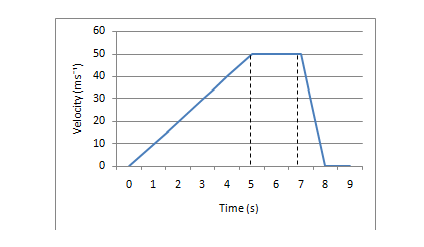Figure 2.1.5 – Velocity -Time graph

Determine its acceleration
We know that the gradient of a velocity- Time graph gives us its acceleration. Therefore for the first 5 s the acceleration is:

50/5 =10 msË²

When the object is at constant speed from 5s to 7s its acceleration is zero. During the last second of the objects journey the object is decelerating at:

50/1 =50 msË²

Determine its displacement
The area under a velocity-time graph is the displacement. During the first 5 s the object has travelled:

½ x 5 x 50 = 125m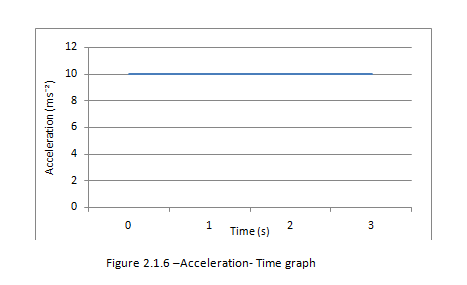Figure 2.1.6 – Acceleration - Time graph

Determine the change in velocity
The area under the acceleration- Time graph gives us the change in velocity

From the graph we find that the change in velocity is 10 x 3

= 30 msË¹

Note: The gradient of the acceleration - time graph is actually the rate of change of acceleration. However it isn’t often useful.

### Forces and Dynamics

2.2.1 Calculate the weight of a body using the expression W = mg.

In general terms one can describe any force as 'the cause of a deformation or a velocity change'. A force is a vector quantity and the SI units for the measurement of force are Newton’s (N).

Weight
The weight of a body is the gravitational force experienced by that body. On earth the formula give is:

W = mg

- The mass of the body measured in kilograms (kg)

g - the gravitational field strength of the earth which is measured in Newton’s per kilogram (N kg-1) or sometimes m s-2. On the surface of the earth g= 9.81 N kg -1.

## 2.2.2 Identify the forces acting on an object and draw free-body diagrams representing the forces acting.

Tension
A string that is taut is said to be under tension. Therefore we can say tension (T) is the force that arises in any body when it is stretched. (Note that a string or rope that is not taut has zero tension in it).Figure 2.2.1 - Tension forces

Normal Reaction force:

If a body touches another body, there is a reaction force(R) between the two bodies. This force is perpendicular to the body exerting the force:Figure 2.2.2 - Normal reaction forces

Drag Force:

Drag forces are forces that oppose the motion of a body through a fluid (gas or liquid). They are directed opposite to the velocity of the body and generally depend on the speed of that body. Higher speed equals higher drag force.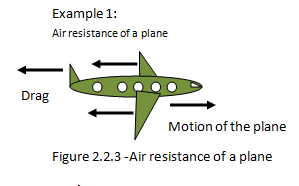Figure 2.2.3 -Air resistance of a plane

Up thrust:
An object placed in a fluid medium will experience up thrust. If the up thrust force on a body is equal to the weight, the body will float in the fluid.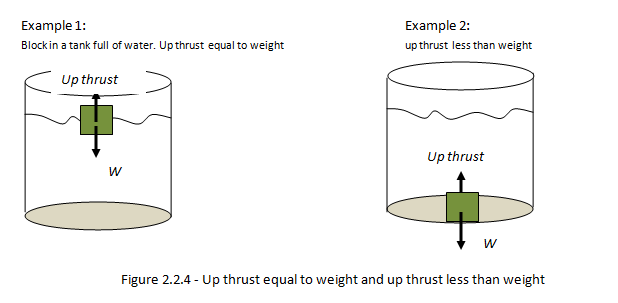Figure 2.2.4 - Up thrust equal to weight and up thrust less than weight

Frictional Forces:
Frictional forces (f) are forces that oppose the motion of a body f.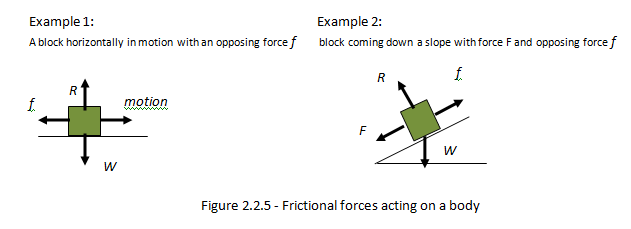Figure 2.2.5 - Frictional forces acting on a body

Hooke’s Law:

Hooke’s law states that up to the elastic limit, the extensionof a spring is proportional to thetension force, F. The constant of proportionality k is called the spring constant. SI units of spring constant are N m-1.

Mathematically,

F∞x

F = Kx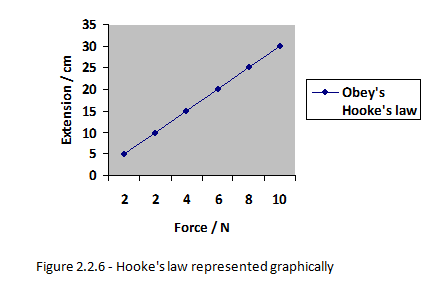Figure 2.2.6 - Hooke's law represented graphically

## 2.2.3 Determine the resultant force in different situations.

The resultant force is the overall force acting on an object when all the individual forces acting on that object have been added together.

In order to determine the resultant force acting upon an object, we need to add the individual forces. Consider figure 2.2.7 below: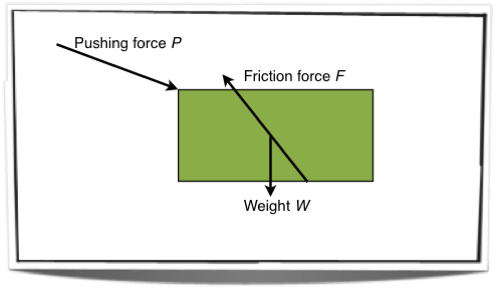Figure 2.2.7 - Forces acting on an object

Figure 2.2.7 shows the free body diagram of an object with three forces acting upon it. In order to determine the resultant force we must add these forces together. This is shown in figure 2.2.8 below: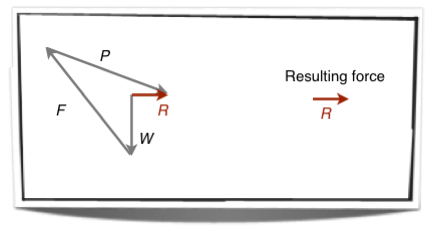Figure 2.2.8 - The resultant force

Figure 2.2.8 shows the resultant force attained through simple vector addition, for more on vector addition see section 1.3.2.

#### Submit Your Assignment/Home work1AssignmentWork Payment (USD)
Content